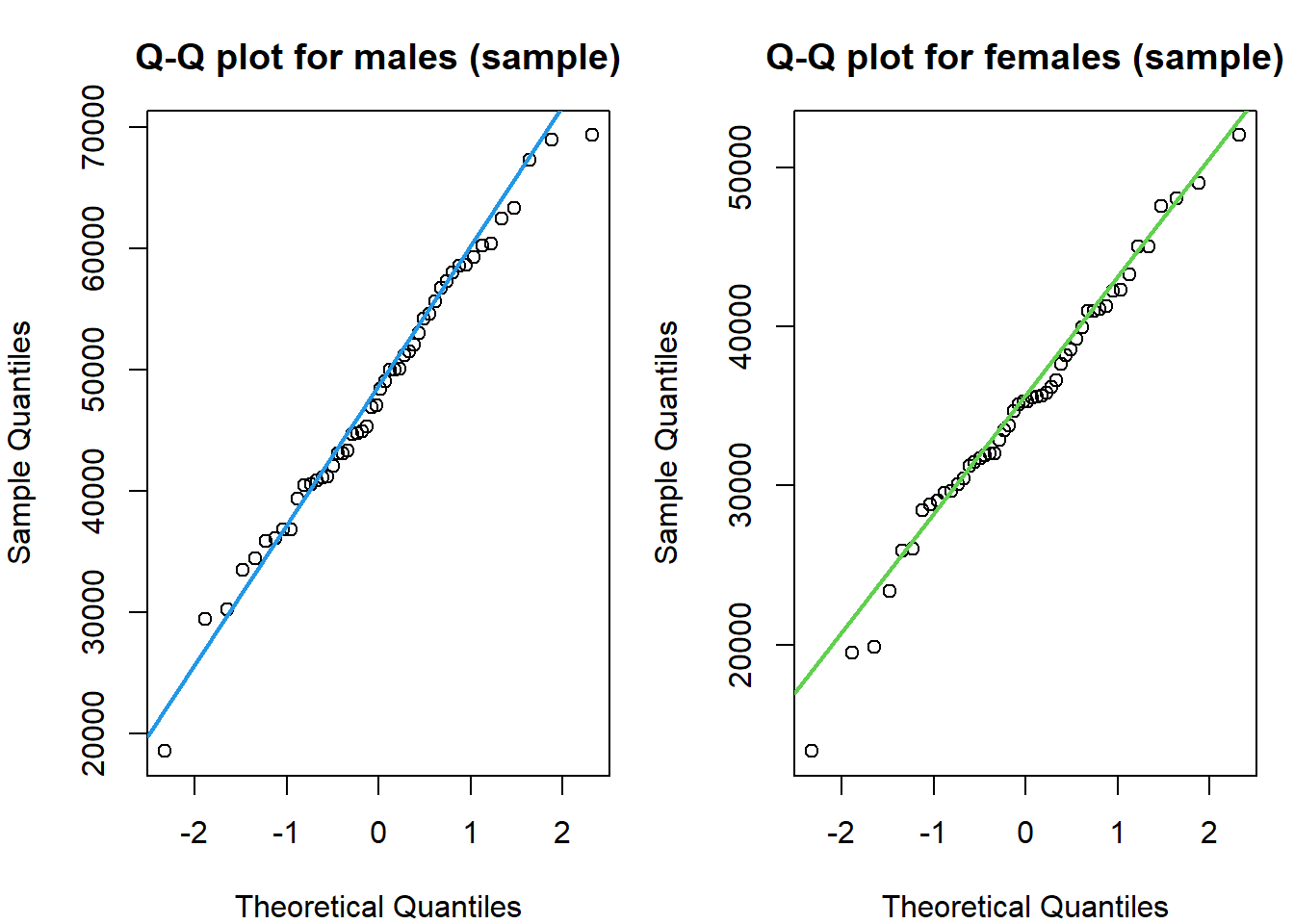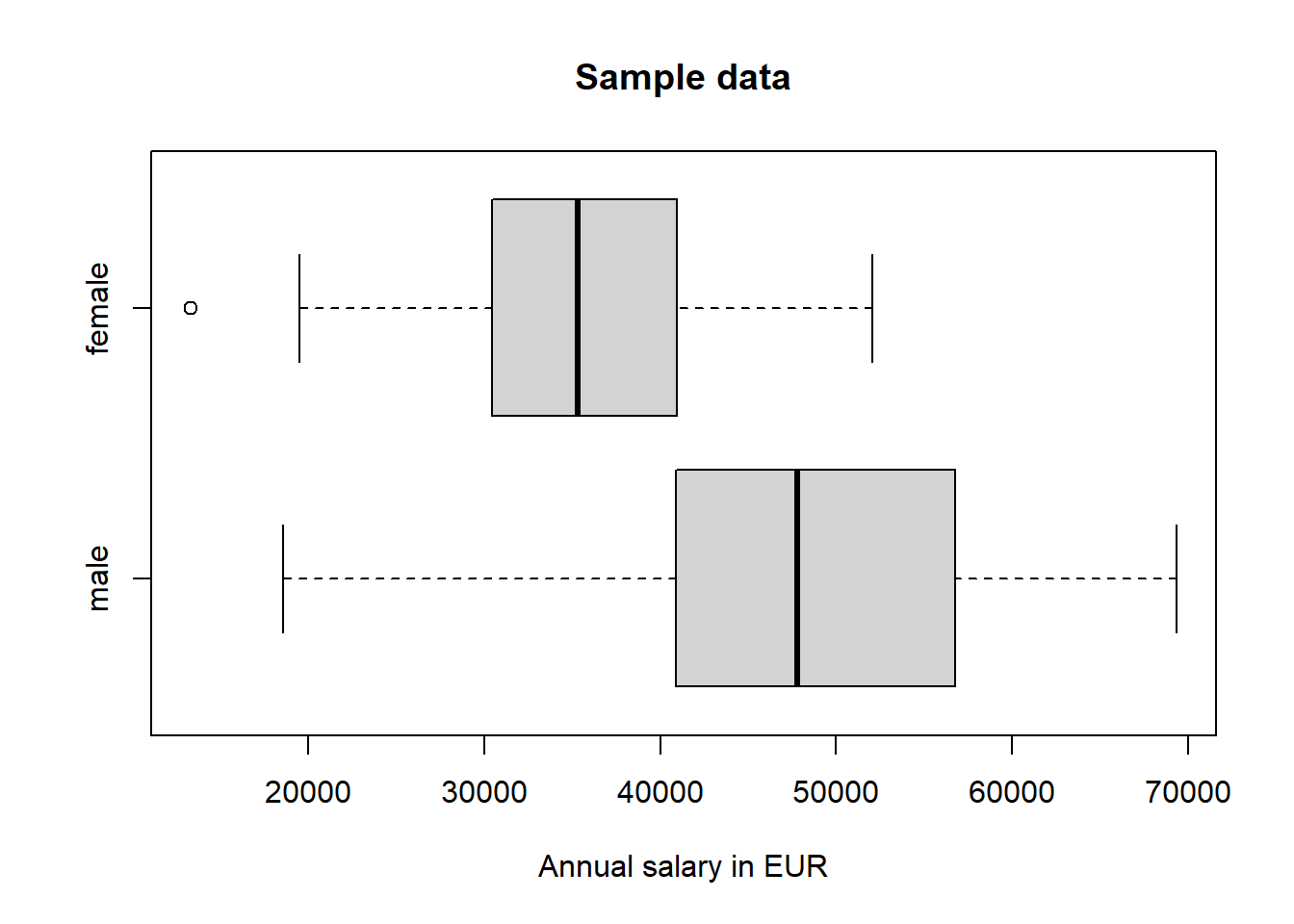In this section we perform a hypothesis test for the means of two populations. We assume that the standard deviations of the two populations are equal but unknown. If, however, we knew $$\sigma$$ and the difference of the sample means ($$\bar x_1 - \bar x_2$$), the test statistic could be written as follows:

$z = \frac{(\bar x_1 - \bar x_2)-(\mu_1-\mu_2)}{\sigma \sqrt{\frac{1}{n_1} + \frac{1}{n_2}}}$

However, in almost all real life applications we do not know $$\sigma$$. Thus, we estimate it beforehand. The best way to do that is to consider the sample variances, $$s^2_1$$ and $$s^2_2$$, as two estimates for $$\sigma^2$$. By pooling the two sample variances and weighting them according to the sample size, the estimate for $$\sigma^2$$ is given by

$s_p^2 = \frac{(n_1-1)s_1^2 + (n_2-1)s_2^2 }{n_1+n_2-2}\text{,}$

and by taking the square root we get

$s_p = \sqrt{\frac{(n_1-1)s_1^2 + (n_2-1)s_2^2 }{n_1+n_2-2}}\text{.}$

The quantity $$s_p$$ is called the pooled sample standard deviation, where the subscript $$p$$ stands for pooled.

The replacement of $$\sigma$$ in the equation above with its estimate $$s_p$$ results in

$t = \frac{(\bar x_1 - \bar x_2)-(\mu_1-\mu_2)}{s_p \sqrt{\frac{1}{n_1} + \frac{1}{n_2}}}$

The denominator of the equation, $$s_p \sqrt{\frac{1}{n_1} + \frac{1}{n_2}}$$, is the estimator of the standard deviation of $$\bar x_1 - \bar x_2$$, which can be written as

$s_{\bar x_1 - \bar x_2} = s_p \sqrt{\frac{1}{n_1} + \frac{1}{n_2}}$

Please note, that the equation for the test statistic $$t$$ has a t-distribution. The degrees of freedom $$(df)$$ are given by

$df = n_1+n_2-2$

#### Interval Estimation of $$\mu_1 - \mu_2$$

The $$100(1-\alpha)$$ % confidence interval for $$\mu_1 - \mu_2$$ is given by

$(\bar x_1 - \bar x_2) \pm t \times s_p \sqrt{\frac{1}{n_1}+\frac{1}{n_2}}\text{,}$ where the value of $$t$$ is obtained from the t-distribution for the given confidence level and $$n_1+n_2-2$$ degrees of freedom.

#### The pooled t-test: An example

The R machinery allows us to conduct a pooled t-test by extending the t.test() function, which we already applied for a one-mean t-test, by adding the argument var.equal = TRUE to the function call.

In order to practice the pooled t-test we load the students data set. You may download the students.csv file here or import the data set directly into R:

students <- read.csv("https://userpage.fu-berlin.de/soga/data/raw-data/students.csv")

The students data set consists of 8239 rows, each of them representing a particular student, and 16 columns, each of them corresponding to a variable/feature related to that particular student. These self-explaining variables are: stud.id, name, gender, age, height, weight, religion, nc.score, semester, major, minor, score1, score2, online.tutorial, graduated, salary.

In order to showcase the pooled t-test we examine the mean annual salary (in Euro) of graduates. The first population consist of male students and the second population of female students. The question is, whether there is a difference in the mean annual salary of graduates related to gender.

#### Data preparation

• We subset the data set based on the binary graduated variable, which indicates if the student has graduated yet. The integer $$1$$ stands for graduated, $$0$$ indicates that the student did not graduate yet.
• We split the data set based on gender (male and female).
• We sample from each subset 50 female and 50 male students and extract the variable of interest, the mean annual salary (in Euro), which is stored in the column salary. We assign those two vectors to the variables male_sample and female_sample.
graduates <- subset(students, graduated == 1)
male <- subset(graduates, gender == "Male")
female <- subset(graduates, gender == "Female")

n <- 50
male_sample <- sample(male$salary, n) female_sample <- sample(female$salary, n)

Further, we test the normality assumption by plotting a normal probability plot, often referred to as Q-Q plot. If the variable is normally distributed, the normal probability plot should be roughly linear.

In R we apply the qqnorm() and the qqline() functions for plotting Q-Q plots.

par(mfrow = c(1, 2), mar = c(4, 4, 3, 1))

qqnorm(male_sample, main = "Q-Q plot for males (sample)")
qqline(male_sample, col = 4, lwd = 2)

qqnorm(female_sample, main = "Q-Q plot for females (sample)")
qqline(female_sample, col = 3, lwd = 2)We see that the sample data is somehow noisy, but it is still roughly normally distributed. The deviations from the straight line in the upper and lower parts suggest, that the probability distribution is slightly skewed.

Further, we check if the standard deviations of the two populations are roughly equal. As a rule of thumb, the condition of equal population standard deviations is met, if the ratio of the larger to the smaller sample standard deviation is less than 2 (Weiss, 2010). Let us assume, that the data of the students data set is a good approximation for the population.

# calculate standard deviations
sd(male$salary) ##  9657.666 sd(female$salary)
##  7729.226
# calculate ratio
sd(male$salary) / sd(female$salary)
##  1.2495

The ratio is approximately 1.249 and thus, we conclude that the equal population standard deviations criterion is fulfilled. A simple visualization technique for evaluating the spread of a variable is to plot a box plot.

boxplot(male_sample,
female_sample,
horizontal = T,
names = c("male", "female"),
xlab = "Annual salary in EUR",
main = "Sample data"
)#### Hypothesis testing

We conduct the pooled t-test by following the step-wise implementation procedure for hypothesis testing.

Step 1: State the null hypothesis $$H_0$$ and alternative hypothesis $$H_A$$

The null hypothesis states that the average annual salary of male graduates ($$\mu_1$$) is equal to the average annual salary of female graduates ($$\mu_2$$).

$H_0: \quad \mu_1 = \mu_2$

Recall, that the formulation of the alternative hypothesis dictates, whether we apply a two-sided, a left-tailed or a right-tailed hypothesis test. We want to test, if the salary of male graduates ($$\mu_1$$) is higher than the average annual salary of female graduates ($$\mu_2$$). Thus, the alternative hypothesis is formulated as follows:

$H_A: \quad \mu_1 > \mu_2$

This formulation results in a right-tailed hypothesis test.

Step 2: Decide on the significance level, $$\alpha$$

$\alpha = 0.01$

alpha <- 0.01

Step 3 and 4: Compute the value of the test statistic and the p-value

For illustration purposes we compute the test statistic manually in R. Recall the equation for the test statistic from above:

$t = \frac{(\bar x_1 - \bar x_2)-(\mu_1-\mu_2)}{s_p \sqrt{\frac{1}{n_1} + \frac{1}{n_2}}}$ If $$H_0$$ is true, then $$\mu_1-\mu_2 =0$$ and thus, the equation simplifies to

$t = \frac{(\bar x_1 - \bar x_2)}{s_p \sqrt{\frac{1}{n_1} + \frac{1}{n_2}}}\text{,}$

where $$s_p$$ is

$s_p = \sqrt{\frac{(n_1-1)s_1^2 + (n_2-1)s_2^2 }{n_1+n_2-2}}$

# compute the value of the test statistic
n1 <- length(male_sample)
n2 <- length(female_sample)
s1 <- sd(male_sample)
s2 <- sd(female_sample)
x1_bar <- mean(male_sample)
x2_bar <- mean(female_sample)

sp <- sqrt(((n1 - 1) * s1^2 + (n2 - 1) * s2^2) / (n1 + n2 - 2))
t <- (x1_bar - x2_bar) / (sp * sqrt(1 / n1 + 1 / n2))
t
##  6.869095

The numerical value of the test statistic is 6.8690952.

In order to calculate the p-value we apply the pt() function. Recall how to calculate the degrees of freedom:

$df = n_1+n_2-2 = 50 + 50 - 2 = 98$

# compute the p-value
df <- n1 + n2 - 2
p <- pt(t, df = df, lower.tail = FALSE)
p
##  2.989766e-10

$$p = 2.9897663\times 10^{-10}$$.

Step 5: If $$p \le \alpha$$, reject $$H_0$$; otherwise, do not reject $$H_0$$

p <= alpha
##  TRUE

The p-value is less than the specified significance level of 0.01; we reject $$H_0$$. The test results are statistically significant at the 1 % level and provide very strong evidence against the null hypothesis.

Step 6: Interpret the result of the hypothesis test

At the 1 % significance level, the data provides very strong evidence to conclude that the average salary of male graduates is higher than the average salary of female graduates.

#### Hypothesis testing in R

We just manually completed a pooled t-test in R. However, please note that we can make use of the full power of the R machinery to obtain the same result as above in just one line of code!

In order to conduct a pooled t-test in R we apply the t.test() function. We provide two vectors as data input. Further, we set var.equal = TRUE to explicitly state, that we apply the pooled version of the t-test. We set the alternative argument to alternative = 'greater', in order to reflect $$H_A: \; \mu_1 >\mu_2$$.

t.test(x = male_sample, y = female_sample, var.equal = TRUE, alternative = "greater")
##
##  Two Sample t-test
##
## data:  male_sample and female_sample
## t = 6.8691, df = 98, p-value = 2.99e-10
## alternative hypothesis: true difference in means is greater than 0
## 95 percent confidence interval:
##  9837.085      Inf
## sample estimates:
## mean of x mean of y
##  48035.52  35062.25

Great success! Compare the output of the t.test() function with our result from above. They match perfectly! Again, we may conclude that at the 1 % significance level, the data provides very strong evidence to conclude that the average salary of male graduates is higher than the average salary of female graduates.

Citation

The E-Learning project SOGA-R was developed at the Department of Earth Sciences by Kai Hartmann, Joachim Krois and Annette Rudolph. You can reach us via mail by soga[at]zedat.fu-berlin.de.You may use this project freely under the Creative Commons Attribution-ShareAlike 4.0 International License.

Please cite as follow: Hartmann, K., Krois, J., Rudolph, A. (2023): Statistics and Geodata Analysis using R (SOGA-R). Department of Earth Sciences, Freie Universitaet Berlin.